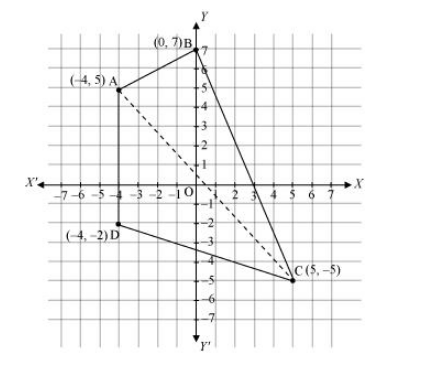# Draw a quadrilateral in the Cartesian plane,

Question:

Draw a quadrilateral in the Cartesian plane, whose vertices are (–4, 5), (0, 7), (5, –5) and (–4, –2). Also, find its area.

Solution:

Let ABCD be the given quadrilateral with vertices A (–4, 5), B (0, 7), C (5, –5), and D (–4, –2).

Then, by plotting A, B, C, and D on the Cartesian plane and joining AB, BC, CD, and DA, the given quadrilateral can be drawn asTo find the area of quadrilateral ABCD, we draw one diagonal, say AC.

Accordingly, area (ABCD) = area (ΔABC) + area (ΔACD)

$=\frac{1}{2}|-4(7+5)+0(-5-5)+5(5-7)|$ unit $^{2}$

$=\frac{1}{2}|-4(12)+5(-2)|$ unit $^{2}$

$=\frac{1}{2}|-48-10|$ unit $^{2}$

$=\frac{1}{2}|-58|$ unit $^{2}$

$=\frac{1}{2} \times 58$ unit $^{2}$

$=29$ unit $^{2}$

Area of $\triangle \mathrm{ACD}$

$=\frac{1}{2}|-4(-5+2)+5(-2-5)+(-4)(5+5)|$ unit $^{2}$

$=\frac{1}{2}|-4(-3)+5(-7)-4(10)|$ unit $^{2}$

$=\frac{1}{2}|12-35-40|$ unit $^{2}$

$=\frac{1}{2}|-63|$ unit $^{2}$

$=\frac{63}{2}$ unit $^{2}$

Thus, area $(\mathrm{ABCD})=\left(29+\frac{63}{2}\right)$ unit $^{2}=\frac{58+63}{2}$ unit $^{2}=\frac{121}{2}$ unit $^{2}$